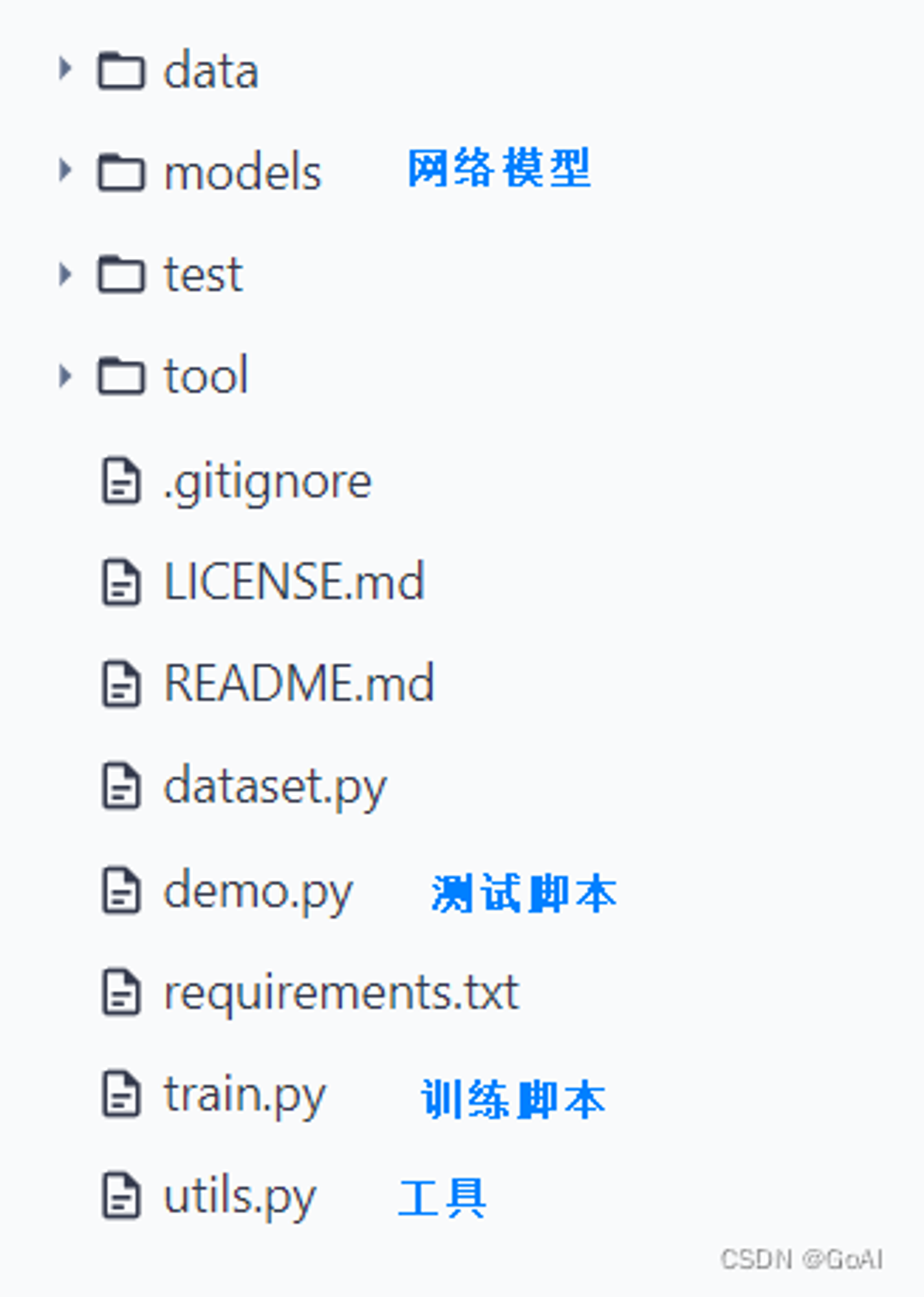GoAI

V1

2023/05/24阅读：16主题：全栈蓝

# OCR项目实战：手写汉语拼音识别

👨‍💻作者简介： 大数据专业硕士在读，CSDN人工智能领域博客专家，阿里云专家博主，专注大数据与人工智能知识分享。

🎉公众号： GoAI的学习小屋，免费分享书籍、简历、导图等资料，更有学习交流群分享AI和大数据资料，方式见文末或主页左侧扫码或私信皆可。

📝OCR专栏导读： 本系列主要介绍计算机视觉领域OCR文字识别领域技术发展方向，每章将分别从OCR技术发展、概念、算法、论文、数据集、现有平台及未来发展方向等各种角度展开详细介绍，综合基础与实战知识。以下是本系列目录，内容目前包括不限于文字检测、识别、表格分析等方向，未来会结合更新NLP方向知识，主要面向深度学习及CV的同学学习，如有错误请大家在评论区指正，如有侵权联系删除。 [ 欢迎添加评论区交流群，群内将分享更多大数据与人工智能方向资料。]

## 「📝OCR入门教程系列目录：」

1️⃣OCR系列第一章 【OCR技术导论】：OCR文字识别技术总结（一） [试读] 2️⃣OCR系列第二章 【OCR基础介绍】：OCR文字识别技术总结（二） 3️⃣OCR系列第三章 【文字检测技术】：OCR文字识别技术总结（三） 4️⃣OCR系列第四章 【文字识别技术】：OCR文字识别技术总结（四） 5️⃣OCR系列第五章 【实战代码解析】：OCR文字识别技术总结（五） 6️⃣OCR系列文章【OCR数据集与评价指标详解】（待更新） 7️⃣OCR系列文章【OCR后处理：文本纠错】（待更新） 8️⃣OCR系列文章 【OCR后处理：版面分析】（待更新） 9️⃣OCR系列文章 【表格识别】（待更新） 🔟OCR系列文章 【关键信息抽取】（待更新） 🆙OCR系列文章 【OCR资料总结】（待更新）

### 📝OCR领域经典论文汇总：

1️⃣OCR文字识别经典论文详解 [试读] 2️⃣OCR文字识别方法综述 3️⃣场景识别文字识别综述（待更新） 4️⃣文字检测方法综述（待更新）

### 📝OCR领域论文详解系列：

1️⃣CRNN：CRNN文字识别 [试读] 2️⃣ASTER：ASTER文本识别详解

🆙目前在整理阶段，后续会更新其他文字检测与识别方向论文解读。

### 📝OCR项目实战系列：

1️⃣Pyotch、TensoFlow

1. 基于CRNN的文本字符交易验证码识别
2. 车牌检测与识别
3. 体检报告识别
4. 中文场景文字识别
5. 手写汉语拼音识别（已更新）
6. 手写英文识别（待更新）
7. 票据识别（待更新）
8. 公式识别（待更新）
9. 表格识别（待更新） ...

****注：更多实战项目敬请期待，详细介绍可以参考本系列其他文章，每个系列对应部分会陆续更新，欢迎大家交流订阅！！

# OCR项目实战（一）：手写汉语拼音识别

## 一、项目介绍：

### 1.项目链接：

https://github.com/GoAlers/Pinyin_recognize 正在整理，后续会补充！

### 2.「项目大致流程：」

1.首先将制作好的图片放入data目录下，图片名按具体写的拼音命名，格式jpg。 2.执行pic_to_txt.py文件，生成all_pic.txt，内容需要包含图片路径名+拼音，空格分割。 3.运行split.py数据集脚本将图片总数量按9:1比例 (将all_pic.txt分别生成train.txt 和test.txt) 4.将txt格式转为lmdb格式数据集执行create_lmdb，得到train和testd lmdb文件夹，将两个路径替换main.py里的训练及测试路径。 5.运行train.py训练，跑一定时间将模型保存运行demo进行测试。

### 二、数据集构建：

#### 数据集格式：

``D:\Python\PycharmProjects\CRNN\ATT_PY\data\bàocháng.png bàochángD:\Python\PycharmProjects\CRNN\ATT_PY\data\diànnǎo.png diànnǎoD:\Python\PycharmProjects\CRNN\ATT_PY\data\guòyǐn.png guòyǐnD:\Python\PycharmProjects\CRNN\ATT_PY\data\húshōuzhàn.png húshōuzhànD:\Python\PycharmProjects\CRNN\ATT_PY\data\kǒngpà.png kǒngpàD:\Python\PycharmProjects\CRNN\ATT_PY\data\péiyǎng.png péiyǎngD:\Python\PycharmProjects\CRNN\ATT_PY\data\qǔdāo.png qǔdāoD:\Python\PycharmProjects\CRNN\ATT_PY\data\tiānnèi.png tiānnèi``

### 五、算法介绍及构建

#### 「1.1CRNN算法」

CRNN的全名为Convolutional Recurrent Neural Network，主要是被设计用来对端到端的长宽度不确定的长文本序列加以识别。它就可以实现不用先去对其他任何一类单个的图像文字序列识别进行切割，而是借助将单个的图形文字序列的辨识学习步骤依次转变为一类由时间序列的依赖图形文本序列的辨识的学习过程的问题，进行更高效地基适应于用单一的图像文字序列实现的所有图像文本序列的标识。

CRNN借鉴采用了语音识别建模理论框架中最为常用的一种LSTM+CTC网络的声音建模分析技术方法，输入接收到的从LSTM所提取得到的声音特征，不再意味着完全可以是当前语音领域所常用到的各种声学特征，而是一种由CNN网络中所无法提取到声音的图像特征。CRNN网络实现结合CNN和RNN网络结构，其结构如图3.5所示：

### 1.1.1.CRNN+CTC

#### 六、代码实战train.py

``# encoding: utf-8from __future__ import print_functionfrom __future__ import divisionimport argparseimport randomimport torchimport torch.backends.cudnn as cudnnimport torch.optim as optimimport torch.utils.datafrom torch.autograd import Variableimport numpy as np#from warpctc_pytorch import CTCLossimport osimport utils as utils1import datasetimport hypy_alphabetimport models.crnn as crnnparser = argparse.ArgumentParser()parser.add_argument('--trainRoot', default = r'../ATT_PY/train502', help='path to dataset')parser.add_argument('--valRoot', default = r'../ATT_PY/test502', help='path to dataset')parser.add_argument('--workers', type=int, help='number of demo_data loading workers', default=0)parser.add_argument('--batchSize', type=int, default=4, help='input batch size')parser.add_argument('--imgH', type=int, default=32, help='the height of the input image to network')parser.add_argument('--imgW', type=int, default=300, help='the width of the input image to network')parser.add_argument('--nh', type=int, default=256, help='size of the lstm hidden state')parser.add_argument('--nepoch', type=int, default=200, help='number of epochs to sxmv for')   #迭代次数# TODO(meijieru): epoch -> iterparser.add_argument('--cuda',default=True, action='store_true', help='enables cuda')    #有GPU时--cuda，另加了default=Trueparser.add_argument('--ngpu', type=int, default=1, help='number of GPUs to use')# parser.add_argument('--pretrained', default='', help="path to pretrained model (to continue training)") #预训练模型，可以设置断#parser.add_argument('--pretrained', default=r'./exprpy/netCRNN_188_73.pth', help="path to pretrained model (to continue training)")# parser.add_argument('--alphabet', type=str, default='-1234abcdefghijklmnopqrstuvwxyz')parser.add_argument('--alphabet', type=str, default='abcdefghijklmnopqrstuvwxyzāáǎàōóǒòēéěèīíǐìūúǔùǖǘǚǜ')parser.add_argument('--expr_dir', default='exprpy', help='Where to store samples and models')   #输出模型路径parser.add_argument('--displayInterval', type=int, default=10, help='Interval to be displayed')parser.add_argument('--n_test_disp', type=int, default=10, help='Number of samples to display when test')parser.add_argument('--valInterval', type=int, default=119, help='Interval to be displayed')  #一轮保留一次模型parser.add_argument('--saveInterval', type=int, default=119, help='Interval to be displayed')  #设置多少次迭代保存一次模型parser.add_argument('--lr', type=float, default=0.0001, help='learning rate for Critic, not used by adadealta')parser.add_argument('--beta1', type=float, default=0.5, help='beta1 for adam. default=0.5')# 以下为两个优化器 ，可以选 ，其中下一行设置default=True，等于默认执行文件时加 --adamparser.add_argument('--adam', default=True, help='Whether to use adam (default is rmsprop)')parser.add_argument('--adadelta', action='store_true', help='Whether to use adadelta (default is rmsprop)')parser.add_argument('--keep_ratio', action='store_true', help='whether to keep ratio for image resize')parser.add_argument('--manualSeed', type=int, default=1234, help='reproduce experiemnt')parser.add_argument('--random_sample', action='store_true', default=True, help='whether to sample the dataset with random sampler')#以上参数可以通过opt.名字访问opt = parser.parse_args()#输出各参数内容print(opt)# opt.alphabet = hypy_alphabet.alphabet()# print(opt.alphabet)if not os.path.exists(opt.expr_dir):    os.makedirs(opt.expr_dir)random.seed(opt.manualSeed)np.random.seed(opt.manualSeed)torch.manual_seed(opt.manualSeed)cudnn.benchmark = Trueif torch.cuda.is_available() and not opt.cuda:    print("WARNING: You have a CUDA device, so you should probably run with --cuda")train_dataset = dataset.lmdbDataset(root=opt.trainRoot)assert train_dataset#设置随机采样if not opt.random_sample:    sampler = dataset.randomSequentialSampler(train_dataset, opt.batchSize)else:    sampler = None#加载训练及测试集train_loader = torch.utils.data.DataLoader(    train_dataset, batch_size=opt.batchSize,    shuffle=True, sampler=sampler,    num_workers=int(opt.workers),    collate_fn=dataset.alignCollate(imgH=opt.imgH, imgW=opt.imgW, keep_ratio=opt.keep_ratio))test_dataset = dataset.lmdbDataset(    root=opt.valRoot, transform=dataset.resizeNormalize((opt.imgW, 32)))#分类含空格+Mathorcuo_finalnclass = len(opt.alphabet) + 1nc = 1converter = utils1.strLabelConverter(opt.alphabet)criterion = torch.nn.CTCLoss()# custom weights initialization called on crnndef weights_init(m):    classname = m.__class__.__name__    if classname.find('Conv') != -1:        m.weight.data.normal_(0.0, 0.02)    elif classname.find('BatchNorm') != -1:        m.weight.data.normal_(1.0, 0.02)        m.bias.data.fill_(0)crnn = crnn.CRNN(opt.imgH, nc, nclass, opt.nh)crnn.apply(weights_init)#加载预训练if opt.pretrained != '':    print('loading pretrained model from %s' % opt.pretrained)    # crnn.load_state_dict(torch.load(opt.pretrained))  #预训练模型出现参数报错，将本行改为下列代码执行成功    crnn.load_state_dict({k.replace('module.', ''): v for k, v in torch.load(opt.pretrained).items()})# print(crnn)image = torch.FloatTensor(opt.batchSize, 3, opt.imgH, opt.imgH)text = torch.IntTensor(opt.batchSize * 5)length = torch.IntTensor(opt.batchSize)if opt.cuda:    crnn.cuda()    # 迭代次数或者epoch足够大的时候，我们通常会使用nn.DataParallel函数来用多个GPU来加速训练    crnn = torch.nn.DataParallel(crnn, device_ids=range(opt.ngpu))    image = image.cuda()    criterion = criterion.cuda()image = Variable(image)# print(image)text = Variable(text)# print(text)length = Variable(length)# print(length)# loss averagerloss_avg = utils1.averager()# 两种优化器参数设置setup optimizerif opt.adam:    optimizer = optim.Adam(crnn.parameters(), lr=opt.lr,                           betas=(opt.beta1, 0.999))elif opt.adadelta:    optimizer = optim.Adadelta(crnn.parameters())else:    optimizer = optim.RMSprop(crnn.parameters(), lr=opt.lr)def val(net, dataset, criterion, max_iter=100):    print('Start val')    for p in crnn.parameters():        p.requires_grad = False    net.eval()    data_loader = torch.utils.data.DataLoader(        dataset, shuffle=True, batch_size=opt.batchSize, num_workers=int(opt.workers))    val_iter = iter(data_loader)    i = 0    n_correct = 0    loss_avg = utils1.averager()    max_iter = min(max_iter, len(data_loader))    for i in range(max_iter):        data = val_iter.next()        i += 1        cpu_images, cpu_texts = data        batch_size = cpu_images.size(0)        utils1.loadData(image, cpu_images)        t,l = converter.encode(cpu_texts)        utils1.loadData(text, t)        utils1.loadData(length, l)        preds = crnn(image)        preds_size = Variable(torch.IntTensor([preds.size(0)] * batch_size))        cost = criterion(preds, text, preds_size, length) / batch_size        loss_avg.add(cost)        _, preds = preds.max(2)        # print(preds.size())        # preds = preds.squeeze(2)        preds = preds.transpose(1, 0).contiguous().view(-1)        sim_preds = converter.decode(preds.data, preds_size.data, raw=False)        for pred, target in zip(sim_preds, cpu_texts):            if pred == target.lower():                n_correct += 1    raw_preds = converter.decode(preds.data, preds_size.data, raw=True)[:opt.n_test_disp]    for raw_pred, pred, gt in zip(raw_preds, sim_preds, cpu_texts):        print('%-20s => %-20s, gt: %-20s' % (raw_pred, pred, gt))    accuracy = n_correct / float(max_iter * opt.batchSize)    print('Test loss: %f, accuray: %f' % (loss_avg.val(), accuracy))def trainBatch(net, criterion, optimizer):    data = train_iter.next()    cpu_images, cpu_texts = data    batch_size = cpu_images.size(0)    utils1.loadData(image, cpu_images)    t, l = converter.encode(cpu_texts)    utils1.loadData(text, t)    utils1.loadData(length, l)    preds = crnn(image)    preds_size = Variable(torch.IntTensor([preds.size(0)] * batch_size))#    print(preds.shape)#    print(text.shape)#    print(text.demo_data, length.demo_data)    cost = criterion(preds, text, preds_size, length) / batch_size    crnn.zero_grad()    cost.backward()    optimizer.step()    return costfor epoch in range(opt.nepoch):    train_iter = iter(train_loader)    i = 0    while i < len(train_loader):        for p in crnn.parameters():            p.requires_grad = True        crnn.train()        cost = trainBatch(crnn, criterion, optimizer)        loss_avg.add(cost)        i += 1  #设置保留模型频率，可以设置每次保留最优模型。        if i % opt.displayInterval == 0:            print('[%d/%d][%d/%d] Loss: %f' %                  (epoch, opt.nepoch, i, len(train_loader), loss_avg.val()))            loss_avg.reset()        if i % opt.valInterval == 0:            val(crnn, test_dataset, criterion)        # do checkpointing        if i % opt.saveInterval == 0:            torch.save(                crnn.state_dict(), '{0}/netCRNN_{1}_{2}.pth'.format(opt.expr_dir, epoch, i))``

### 1.1.2.CRNN+Attenton

#### 算法网络结构

• 编码网络系统包括两个由两个单向的ResNet(残差神经网络)和另外两个由双向的LSTM(长短期记忆网络)而构成的。ResNet网络是通过不同的卷积核大小将用户输入到的手写或汉语拼音图像信息进行了多层次的特征化提取，进而获得特征图像，再借助全连接操作将特征图像转化为特征序列。特征序列借助双向LSTM网络转变获得一种固定的宽度的矩阵。

• 解码网络由单向LSTM网络、注意力机制和Softmax组成。单向的LSTM网络主要作用就是把特征序列转化为相应的拼音序列，将目标矩阵上的信息进行训练。注意力机制作为解码编码过程中的重要纽带，它能从编码器获取每一处隐藏信息，从而提供给解码器做进一步处理，使该模型更关注手写汉语拼音特征序列的重要信息。Softmax则是对提取到的特征进行多分类，最后将结果一一提取。

### 九、不足及改进：

1.对于相对清晰的手写汉语拼音图片可以具有很好的识别效果，但是对于一些字迹连笔、字迹很淡的图片，测试效果就并不好，准确度较低，因此，算法还有需要改进的地方。 2.数据集的样本图片数量也相对较少，所以能达到的识别效果有限，如果数据集更加丰富的话，识别效果会更好。另外，在网络模型选择上，本文仅使用CRNN基本模型，vgg网络进行特征提取，后续可以更换ResNet、MoblineNetV3等其他网络进行实验。 3.而且在实验过程中，由于实验设备的配置较低，每次调整参数进行实验都会耗费大量的时间，一些调试中的问题解决起来会比较困难，所以并没有做更多的模型对比实验。 以上这些不足都还需要继续改进，才能更好的应用于手写汉语拼音识别的实现。V1